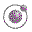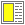The Order of Evaluation - slide 10 : 26

The alpha rewrite rule
An alpha conversion changes the names of lambda expression formal parameters

Formal parameters of a lambda expression can be substituted by other names, which are not used as free names in the body

Legal conversion:
 Expression Converted Expression `(lambda (x y) (f x y))` `(lambda (a b) (f a b))`
Illegal conversion:
 Expression Converted Expression `(lambda (x y) (f x y))` `(lambda (a f) (f a f))`